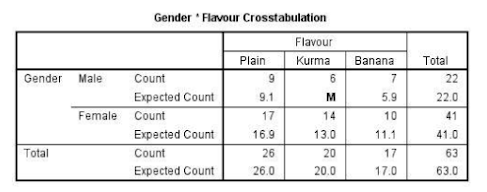Aug 23, 2023

QUESTION 7

A dairy products factory wants to know the milk flavor preferred by the buyers. The researchers randomly selected several supermarket visitors and conducted an experiment. Buyers were given three cups of milk with different flavors to drink. After that, they were asked to choose one flavor that they preferred the most. The data collected are shown in the following table.a) Find the value of M by using the expected value formula.

b) Calculate the value of statistic value for this study.

c) At the 10% significance level, what can you conclude about the relationship between the two variables?

d) If the variables are measured in ratio, can the Chi-Square Test of Independence be used?